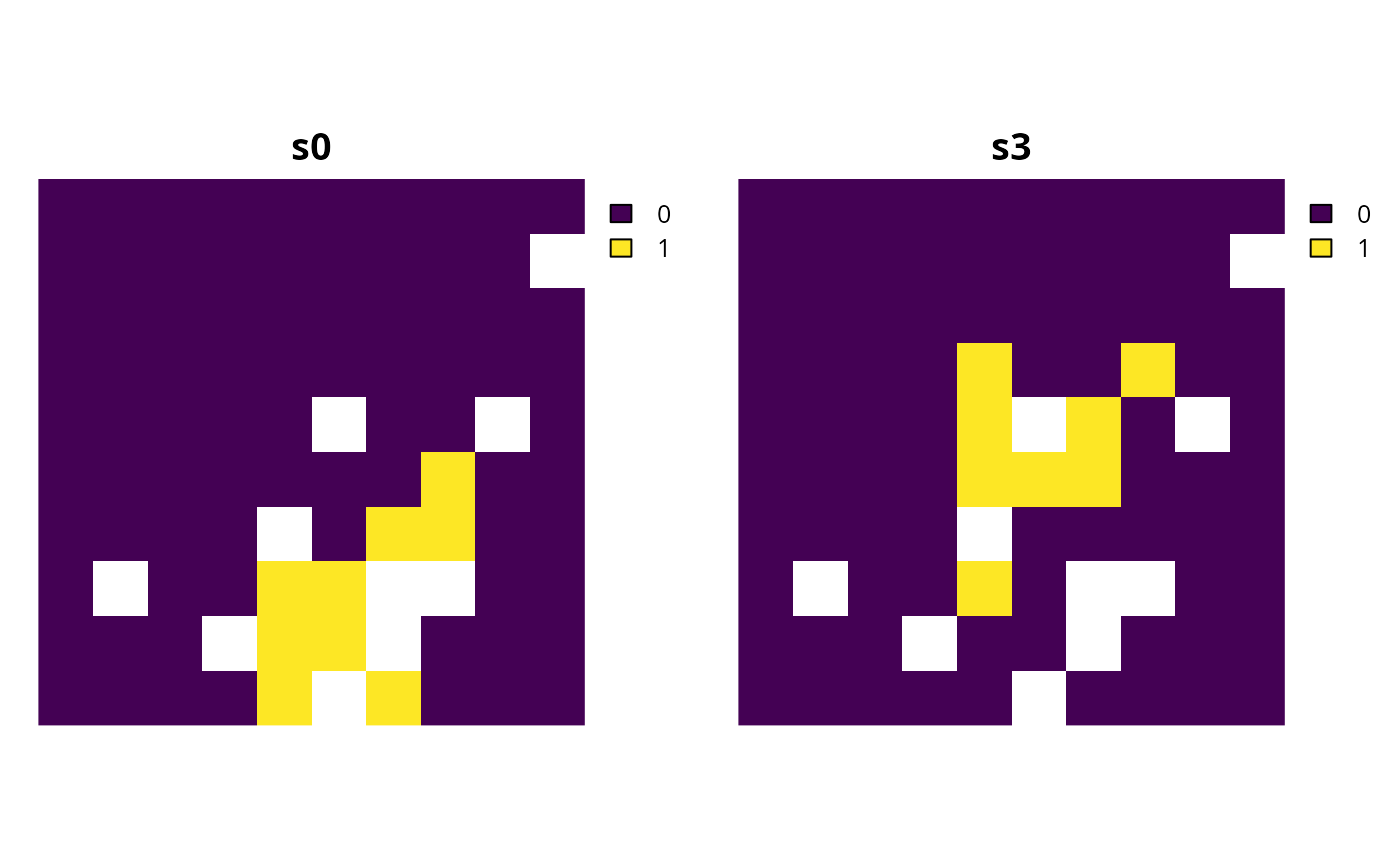Add constraints to a conservation planning problem to ensure that all selected planning units meet certain criteria.

## Usage

# S4 method for ConservationProblem,ANY,ANY,character

# S4 method for ConservationProblem,ANY,ANY,numeric

# S4 method for ConservationProblem,ANY,ANY,matrix

# S4 method for ConservationProblem,ANY,ANY,Matrix

# S4 method for ConservationProblem,ANY,ANY,Raster

# S4 method for ConservationProblem,ANY,ANY,SpatRaster

# S4 method for ConservationProblem,ANY,ANY,dgCMatrix
add_linear_constraints(x, threshold, sense, data)

## Arguments

x

problem() object.

threshold

numeric value. This threshold value is also known as a "right-hand-side" value per integer programming terminology.

sense

character sense for the constraint. Available options include ">=", "<=", or "=" values.

data

character, numeric, terra::rast(), matrix, or Matrix object containing the constraint values. These constraint values are also known as constraint coefficients per integer programming terminology. See the Data format section for more information.

## Value

An updated problem() object with the constraints added to it.

## Details

This function adds general purpose constraints that can be used to ensure that solutions meet certain criteria (see Examples section below for details). For example, these constraints can be used to add multiple budgets. They can also be used to ensure that the total number of planning units allocated to a certain administrative area (e.g., country) does not exceed a certain threshold (e.g., 30% of its total area). Furthermore, they can also be used to ensure that features have a minimal level of representation (e.g., 30%) when using an objective function that aims to enhance feature representation given a budget (e.g., add_min_shortfall_objective()).

## Mathematical formulation

The linear constraints are implemented using the following equation. Let $$I$$ denote the set of planning units (indexed by $$i$$), $$Z$$ the set of management zones (indexed by $$z$$), and $$X_{iz}$$ the decision variable for allocating planning unit $$i$$ to zone $$z$$ (e.g., with binary values indicating if each planning unit is allocated or not). Also, let $$D_{iz}$$ denote the constraint data associated with planning units $$i \in I$$ for zones $$z \in Z$$ (argument to data, if supplied as a matrix object), $$\theta$$ denote the constraint sense (argument to sense, e.g., $$<=$$), and $$t$$ denote the constraint threshold (argument to threshold).

$$\sum_{i}^{I} \sum_{z}^{Z} (D_{iz} \times X_{iz}) \space \theta \space t$$

## Data format

The argument to data can be specified using the following formats.

data as character vector

containing column name(s) that contain penalty values for planning units. This format is only compatible if the planning units in the argument to x are a sf::sf() or data.frame object. The column(s) must have numeric values, and must not contain any missing (NA) values. For problems that contain a single zone, the argument to data must contain a single column name. Otherwise, for problems that contain multiple zones, the argument to data must contain a column name for each zone.

data as a numeric vector

containing values for planning units. These values must not contain any missing (NA) values. Note that this format is only available for planning units that contain a single zone.

data as a matrix/Matrix object

containing numeric values that specify data for each planning unit. Each row corresponds to a planning unit, each column corresponds to a zone, and each cell indicates the data for penalizing a planning unit when it is allocated to a given zone.

data as a terra::rast() object

containing values for planning units. This format is only compatible if the planning units in the argument to x are sf::sf(), or terra::rast() objects. If the planning unit data are a sf::sf() object, then the values are calculated by overlaying the planning units with the argument to data and calculating the sum of the values associated with each planning unit. If the planning unit data are a terra::rast() object, then the values are calculated by extracting the cell values (note that the planning unit data and the argument to data must have exactly the same dimensionality, extent, and missingness). For problems involving multiple zones, the argument to data must contain a layer for each zone.

See constraints for an overview of all functions for adding constraints.

Other constraints: add_contiguity_constraints(), add_feature_contiguity_constraints(), add_locked_in_constraints(), add_locked_out_constraints(), add_mandatory_allocation_constraints(), add_manual_bounded_constraints(), add_manual_locked_constraints(), add_neighbor_constraints()

## Examples

# \dontrun{
sim_pu_raster <- get_sim_pu_raster()
sim_features <- get_sim_features()

# create a baseline problem with minimum shortfall objective
p0 <-
problem(sim_pu_raster, sim_features) %>%

# solve problem
s0 <- solve(p0)

# plot solution
plot(s0, main = "solution", axes = FALSE)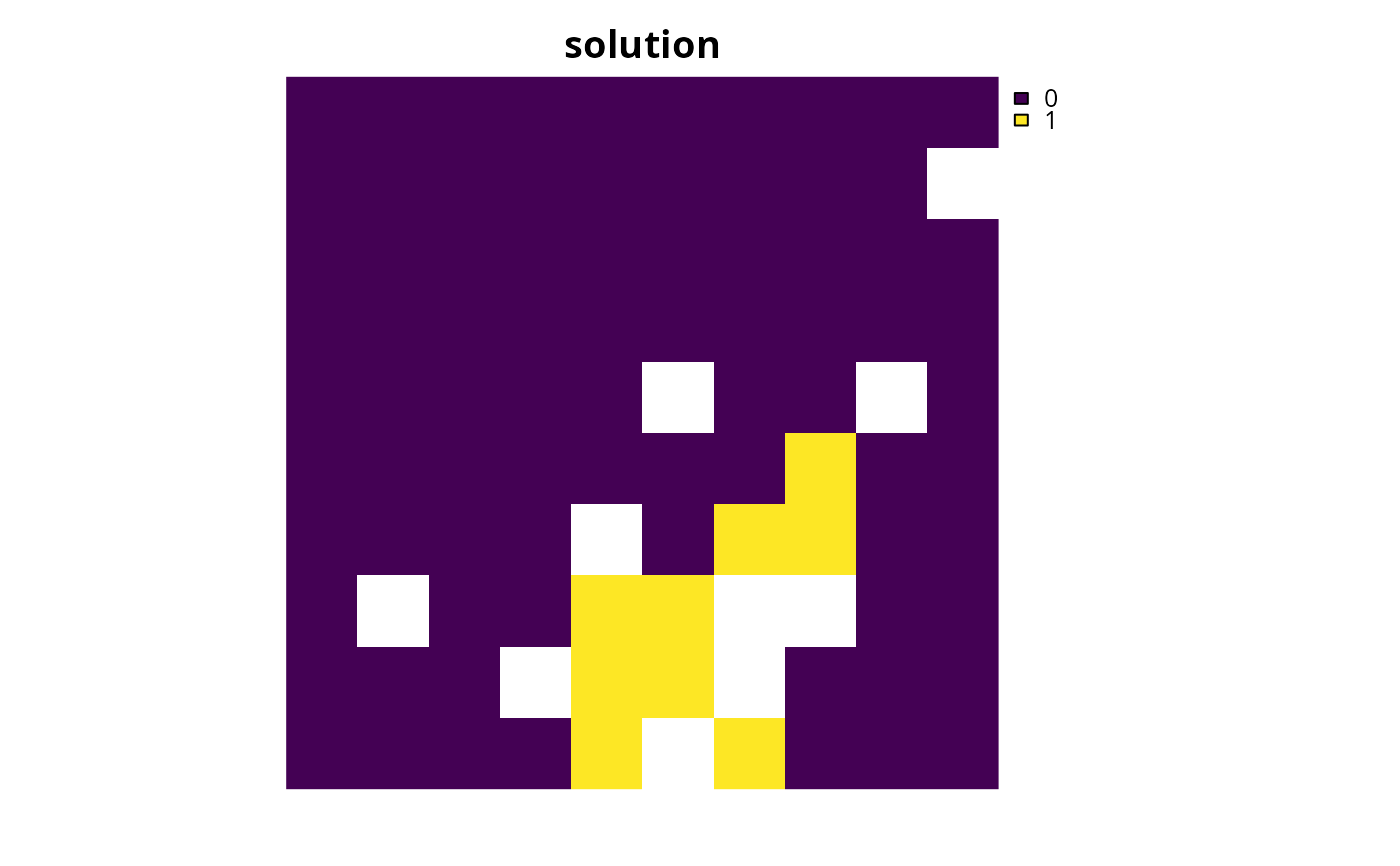# now let's create some modified versions of this baseline problem by

# first, let's create a modified version of p0 that contains
# an additional budget of 1600 based on a secondary cost dataset

# create a secondary cost dataset by simulating values
sim_pu_raster2 <- simulate_cost(sim_pu_raster)

# plot the primary cost dataset (sim_pu_raster) and
# the secondary cost dataset (sim_pu_raster2)
plot(
c(sim_pu_raster, sim_pu_raster2),
main = c("sim_pu_raster", "sim_pu_raster2"),
axes = FALSE
)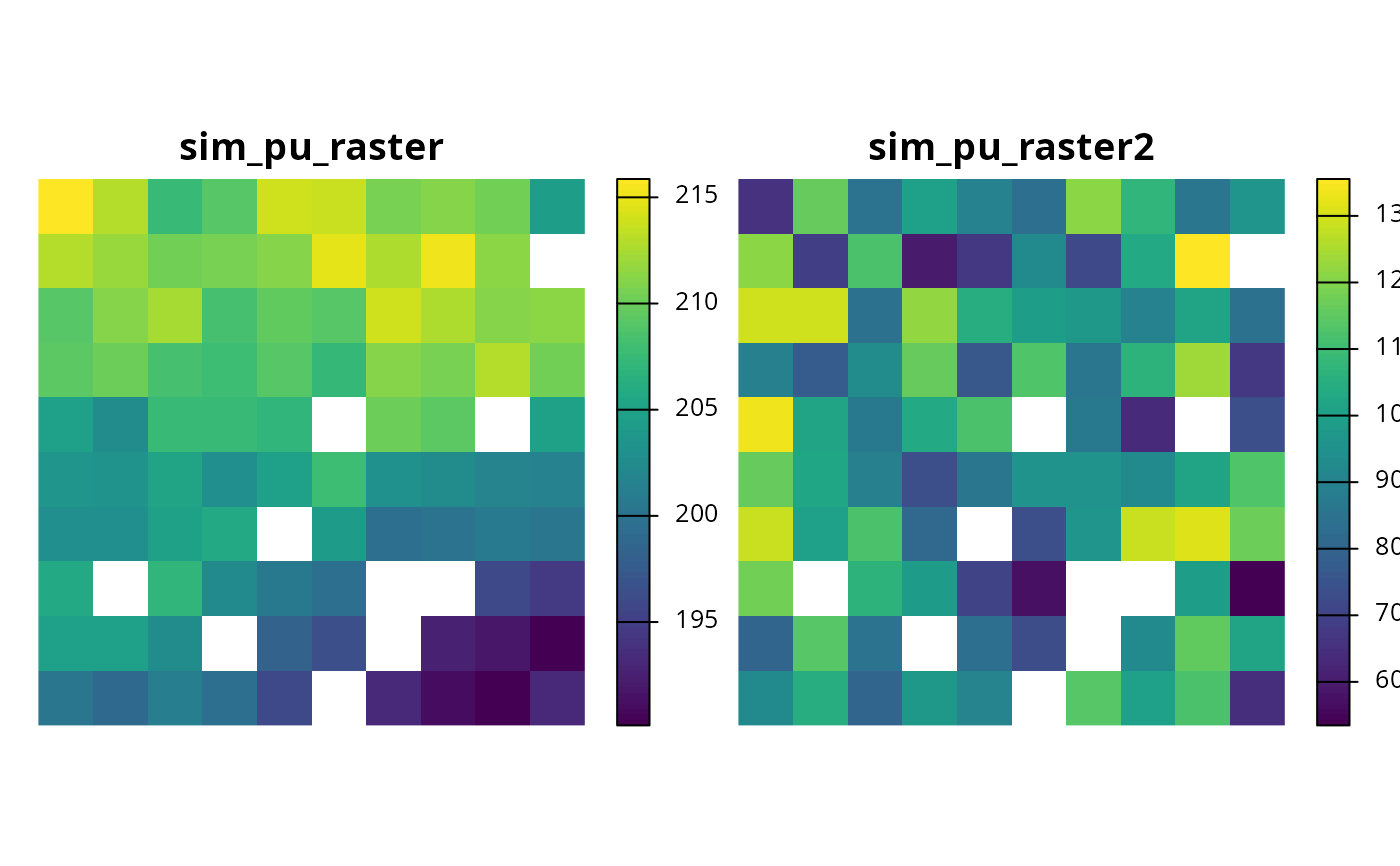# create a modified version of p0 with linear constraints that
# specify that the planning units in the solution must not have
# values in sim_pu_raster2 that sum to a total greater than 500
p1 <-
p0 %>%
threshold = 500, sense = "<=", data = sim_pu_raster2
)

# solve problem
s1 <- solve(p1)

# plot solutions s1 and s2 to compare them
plot(c(s0, s1), main = c("s0", "s1"), axes = FALSE)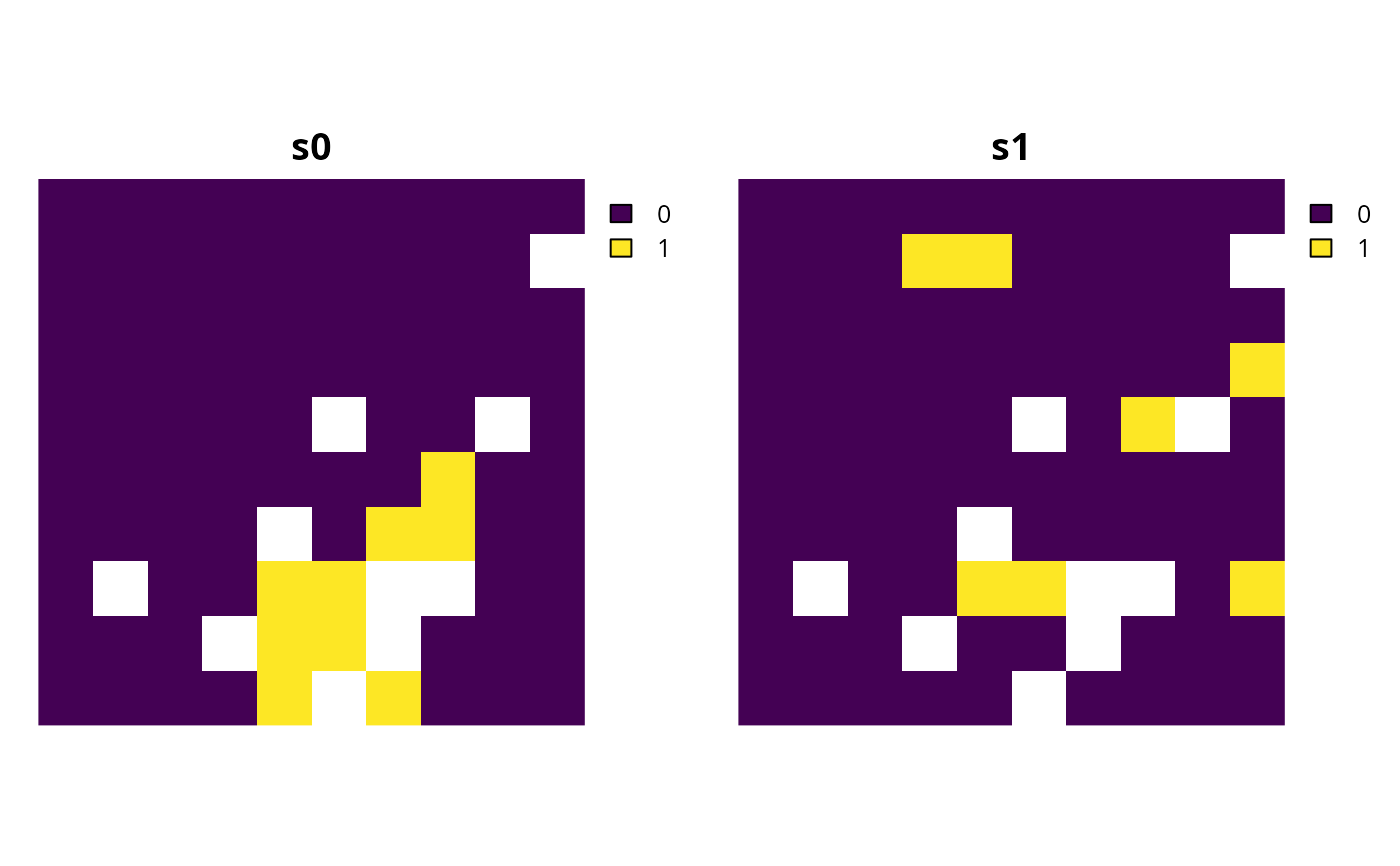# second, let's create a modified version of p0 that contains
# additional constraints to ensure that each feature has
# at least 8% of its overall distribution represented by the solution

# to achieve this, we need to calculate the total amount of each feature
# within the planning units so we can, in turn, set the constraint thresholds
feat_abund <- feature_abundances(p0)\$absolute_abundance

# create a modified version of p0 with additional constraints for each
# feature to specify that the planning units in the solution must
# secure at least 8% of the total abundance for each feature
p2 <- p0
for (i in seq_len(terra::nlyr(sim_features))) {
p2 <-
p2 %>%
threshold = feat_abund[i] * 0.08,
sense = ">=",
data = sim_features[[i]]
)
}

# overall, p2 could be described as an optimization problem
# that maximizes feature representation as much as possible
# towards securing 20% of the total amount of each feature,
# whilst ensuring that (i) the total cost of the solution does
# not exceed 1800 (per cost values in sim_pu_raster) and (ii)
# the solution secures at least 8% of the total amount of each feature
# (if 20% is not possible due to the budget)

# solve problem
s2 <- solve(p2)

# plot solutions s0 and s2 to compare them
plot(c(s0, s2), main = c("s1", "s2"), axes = FALSE)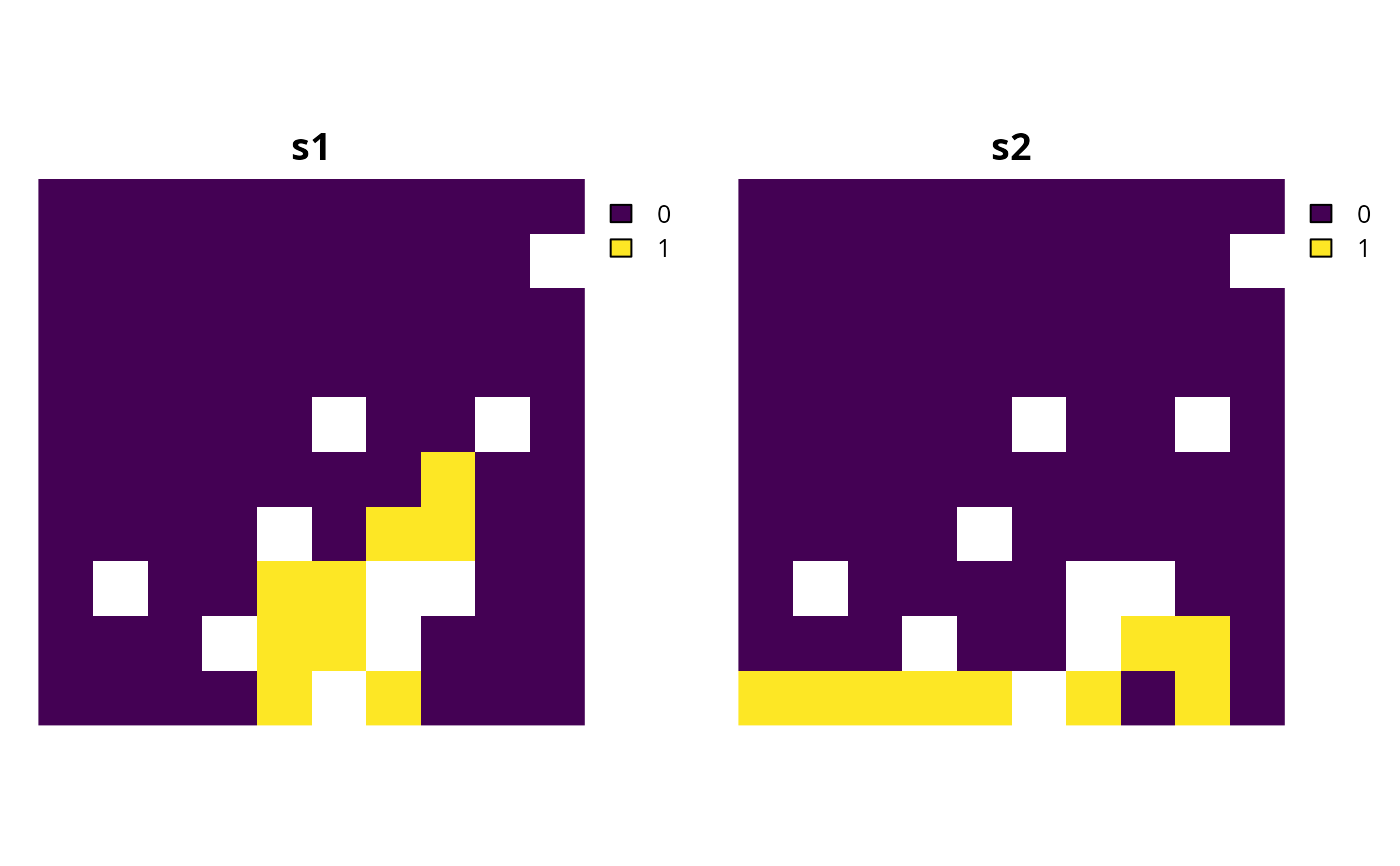# third, let's create a modified version of p0 that contains
# additional constraints to ensure that the solution equitably
# distributes conservation effort across different administrative areas
# (e.g., countries) within the study region

# to begin with, we will simulate a dataset describing the spatial extent of
# four different administrative areas across the study region

# we can see that the administrative areas subdivide
# the study region into four quadrants, and the sim_admin object is a
# SpatRaster with integer values denoting ids for the administrative areas# next we will convert the sim_admin SpatRaster object into a SpatRaster
# object (with a layer for each administrative area) indicating which
# planning units belong to each administrative area using binary
# (presence/absence) values

# plot binary stack of administrative areas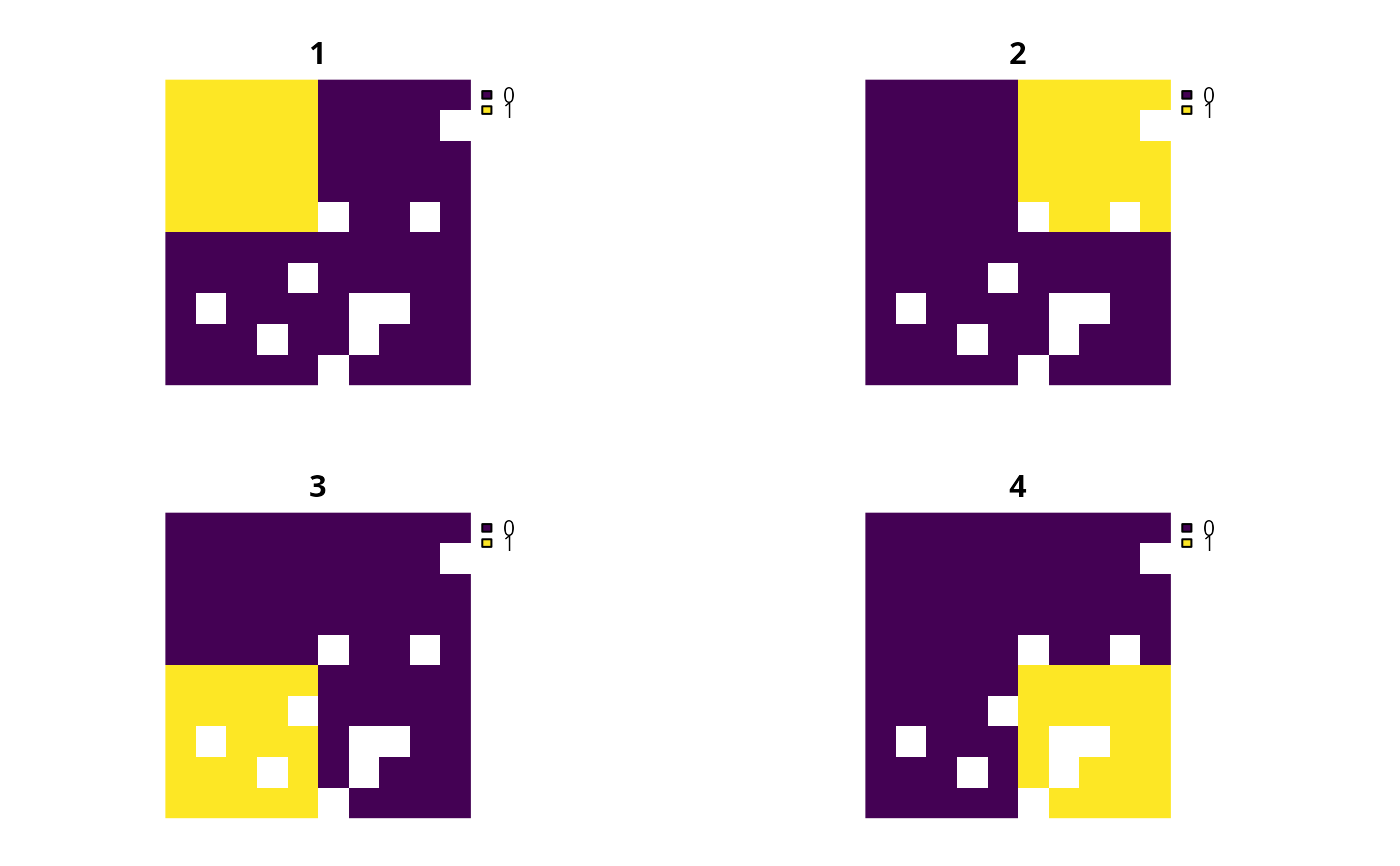# we will now calculate the total amount of planning units associated
# with each administrative area, so that we can set the constraint threshold

# since we are using raster data, we won't bother explicitly
# accounting for the total area of each planning unit (because all
# planning units have the same area in raster formats) -- but if we were
# using vector data then we would need to account for the area of each unit

# create a modified version of p0 with additional constraints for each
# administrative area to specify that the planning units in the solution must
# not encompass more than 10% of the total extent of the administrative
# area
p3 <- p0
p3 <-
p3 %>%
sense = "<=",